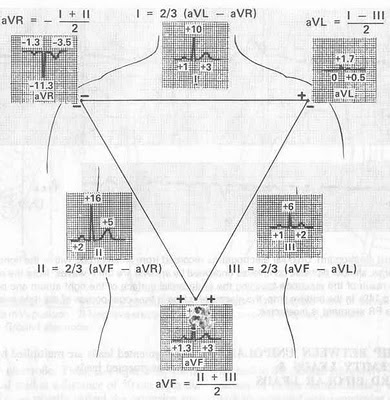## 11/06/2011

### EKG - Normal values, BasicsKirschoff's Law: Algebraic sum of all the potential differences in a closed circuit equals zero. Based on this law, I + II + III = 0.

But, Einthoven reversed the polarity of II. Instead of connecting RA - LL, he connected it LL - RA. Therefore, the formula becomes I - II + III = 0.

When this equation is solved, it becomes Einthoven's equation: II = I + III.

(To verify, take the net amplitude of QRS complexes respective leads from any EKG and do the calculations)

Relationship between Augmented unipolar limb leads (aVR, aVL, aVF) & Standard bipolar leads (I, II, III):
aVR = (I + II)/2
aVL = (I - III)/2
aVF = (II + III)/2

From Principles of clincal electrocardiography by Mervin J. Goldman. 11th ed. 1982. Lange Medical Publications.